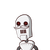# Q.8 Smith and Ajit can complete a task in 12 days and 18 days, respectively. If they work together on the task for 4 days, January 4, 2022 by Kinsley

Q.8 Smith and Ajit can complete a task in 12 days and 18 days, respectively. If they work together on the task for 4 days,
then the fraction of the task that will be left is:

### 1 thought on “<br />Q.8 Smith and Ajit can complete a task in 12 days and 18 days, respectively. If they work together on the task for 4 days,<b”

1.Step-by-step explanation:

Smith can complete a task in 12 days

which means he can compete 1/12 of a work in a day

similarly Ajit’s work in a day is 1/18

and together their work

=1/12 +1/18= (2+3)/36= 5/36

Now if they work for 4 days

= 5/36× 4 = 5/9

therefore task left =1-5/9= 9-5/9= 4/9